# Olympiad Test: Mensuration - 2

## 20 Questions MCQ Test Mathematical Olympiad Class 8 | Olympiad Test: Mensuration - 2

Description
Attempt Olympiad Test: Mensuration - 2 | 20 questions in 40 minutes | Mock test for Class 8 preparation | Free important questions MCQ to study Mathematical Olympiad Class 8 for Class 8 Exam | Download free PDF with solutions
QUESTION: 1

### The radius and height of a cylinder are in the ratio 3 : 7. Its volume is 1584 cu cm. What is the radius of the cylinder?

Solution:

Let radius be 3x and height be 7x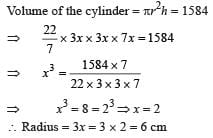QUESTION: 2

### How many bricks of size 22 cm × 10 cm × 7 cm are required to construct a wall 33 m long 3.5 m high and 40 cm thick and sand used in the construction occupy 1/10 th part of the wall?

Solution:

Volume of the wall = 33 × 3.5 × 0.4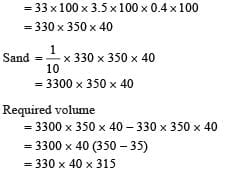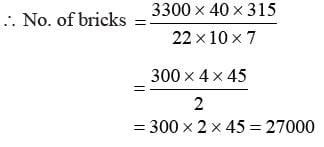QUESTION: 3

### A rectangular sheet of paper 44 cm × 18 cm is rolled along its length and a cylinder is forms. What is the volume of that cylinder?

Solution: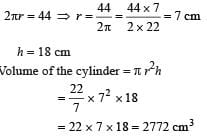QUESTION: 4

The area of the base of a cone is 180 cm2. If the height of the cone is 8 cm. What is its volume?

Solution: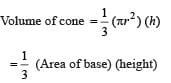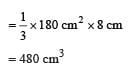QUESTION: 5

The radii of two cylinders are in the ratio 2: 3 and their heights are in the ratio 5 : 3. What is the ratio of their volumes?

Solution:

Given, the radii of cylinder = 2 : 3.
Heights of cylinder = 5 : 3
∴ Ratio of volume of the cylinders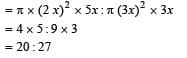QUESTION: 6

A swimming pool is 260 m long and 140 m wide. If 54600 cubic metres of water is pumped into it, what is the height of the water level in it ?

Solution:
QUESTION: 7

A rectangular piece paper 22 cm × 6 cm is folded without overlapping to make a cylinder of height 6 cm. What is the volume of the cylinder?

Solution:

Here h = 6 cm.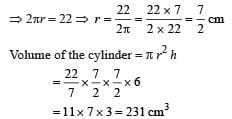QUESTION: 8

The lateral surface area of a cylinder is 11440 cm3. If its height is 65 cm then what is its circumference?

Solution:

Lateral surface area = 11440.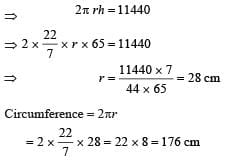QUESTION: 9

In the given figure the outer dimension is 24 cm × 28 cm and the inner dimension 16 cm × 20 cm. What is the difference between two adjacent section of the frame if the width of each section is same?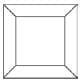Solution:

Width of section AEFB = width of CGHD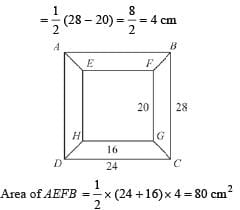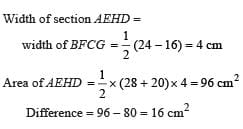QUESTION: 10

The area of a trapezium is 480 cm2. The distance between two parallel sides is 15 m and one of the parallel side is 20 m. What is the length of other parallel side?

Solution: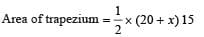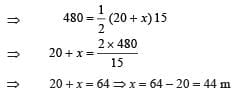QUESTION: 11

A rectangular piece of paper 11 cm × 4 cm is folded without overlapping to make a cylinder of height 4 cm. What is its volume?

Solution:

Here h = 4 cm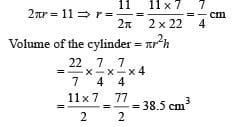QUESTION: 12

The total surface area of a cube is 486 m2. Then what is its side?

Solution:

Total surface area of cube = 486 (Given)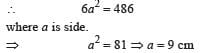QUESTION: 13

The parallel sides of a trapezium are 25 cm and 11 cm and its non-parallel sides are 15 cm and 13 cm. What is the area of trapezium?

Solution:

Here MC = AD = 15 cm
AM = DC = 11 cm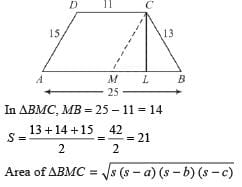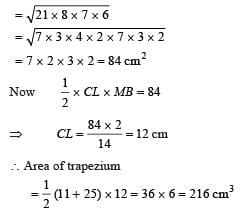QUESTION: 14

The area of a trapezium is 384 cm2. Its parallel sides are in the ratio 5 : 3 and the distance between them is 12 cm. What is the longer of the parallel sides

Solution:

Area of trapezium = 384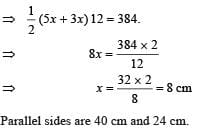QUESTION: 15

In the given figure ST = SR, PQ = QR = RT =TP = 25 m and its 5 total height is 41 m. What is its total area?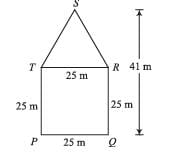Solution:

Total area = area of ΔSTR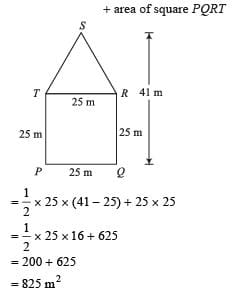QUESTION: 16

ABCD is a quadrilateral field in which the diagonal BD is 36 m. AL ⊥ BD and CM ⊥ BD such that AL = 19 m and CM = 11 m. What is the area of the field?

Solution: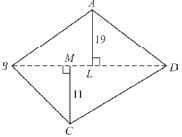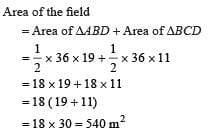QUESTION: 17

If the length of each side of a cube is doubled then how many times does its surface area become?

Solution:

Let the length of cube = a.
Surface area = 6a2.
∴ Length of cube = 2a.
Surface area = 6(2a)2 = 6 × 4a2 = 24a2
= 4 × 6a2

QUESTION: 18

The edges of a cuboid are in the ratio 1 : 2 : 3 and its surface area is 88 cm2. What is the volume of the cuboid?

Solution:

Total surface area of cuboid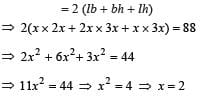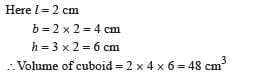QUESTION: 19

The curved surface area of a cylinder is 220 cm2 and the volume of the cylinder is 770 cm3 the what is the diameter of cylinder?

Solution:

Curved surface area of cylinder = 220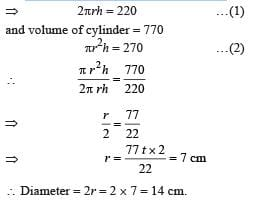QUESTION: 20

The circumference of the circular base of a cylinder is 44 cm and its height is 15 cm. What is the volume of the cylinder?

Solution: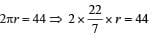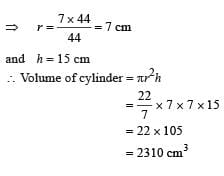Use Code STAYHOME200 and get INR 200 additional OFF Use Coupon Code